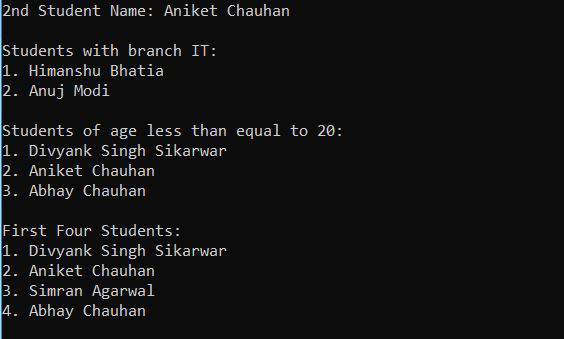# XPath Evaluator in Java

• Difficulty Level : Easy
• Last Updated : 15 Oct, 2021

As we know XML document is used to store and transport data. So, to access data from XML, we need something which could access each node and respective attributes data. Then the solution is XPath. XPath can be used to traverse through XML documents, to select nodes/elements, and attribute data. It is a W3C Recommendation and a flexible way of accessing different parts of an XML document. To write XPath is similar to writing path expression in your computer system to traverse to a specific location like (C:/School/Homework/assignment.docx).

Consider the Following XML Document

Attention reader! Don’t stop learning now. Get hold of all the important Java Foundation and Collections concepts with the Fundamentals of Java and Java Collections Course at a student-friendly price and become industry ready. To complete your preparation from learning a language to DS Algo and many more,  please refer Complete Interview Preparation Course.

## XML

 ```<``students``>``    ``<``student` `branch``=``"CSE"``>``        ``<``name``>Divyank Singh Sikarwar``        ``<``age``>18``        ``<``city``>Agra``    ````    ``<``student` `branch``=``"CSE"``>``        ``<``name``>Aniket Chauhan``        ``<``age``>20``        ``<``city``>Shahjahanpur``    ````    ``<``student` `branch``=``"CSE"``>``        ``<``name``>Simran Agarwal``        ``<``age``>23``        ``<``city``>Buland Shar``    ````    ``<``student` `branch``=``"CSE"``>``        ``<``name``>Abhay Chauhan``        ``<``age``>17``        ``<``city``>Shahjahanpur``    ````    ``<``student` `branch``=``"IT"``>``        ``<``name``>Himanshu Bhatia``        ``<``age``>25``        ``<``city``>Indore``    ````    ``<``student` `branch``=``"IT"``>``        ``<``name``>Anuj Modi``        ``<``age``>22``        ``<``city``>Ahemdabad``    ````    ``<``student` `branch``=``"ECE"``>``        ``<``name``>Manoj Yadav``        ``<``age``>23``        ``<``city``>Kota``    ``  ```

XPath symbols that are used to access different parts of an XML document:

### Let’s Practice XPath

Consider above mentioned XML document:

Select 2nd student

/students/student/name

Select all the students with branch IT

/students/student[@branch = /”IT/”]/name

Select all the students whose age is less than equal to 20

/students/student[age <= 20]/name

First 4 students

/students/student[position() <= 4]/name

## Java

 `import` `java.io.File;``import` `javax.xml.parsers.DocumentBuilder;``import` `javax.xml.parsers.DocumentBuilderFactory;``import` `javax.xml.xpath.XPath;``import` `javax.xml.xpath.XPathConstants;``import` `javax.xml.xpath.XPathExpression;``import` `javax.xml.xpath.XPathExpressionException;``import` `javax.xml.xpath.XPathFactory;``import` `org.w3c.dom.Document;``import` `org.w3c.dom.NodeList;`` ` `public` `class` `XPathDemo {`` ` `    ``public` `static` `void` `main(String[] args) ``throws` `Exception``    ``{``        ``File xmlFile = ``new` `File(``"student.xml"``);``       ` `        ``// Get DOM``        ``DocumentBuilderFactory dbf = DocumentBuilderFactory.newInstance();``        ``DocumentBuilder db = dbf.newDocumentBuilder();``        ``Document xml = db.parse(xmlFile);``        ``xml.getDocumentElement().normalize();`` ` `        ``// Get XPath``        ``XPathFactory xpf = XPathFactory.newInstance();``        ``XPath xpath = xpf.newXPath();`` ` `        ``// Find 2nd Student's name``        ``String name = (String)xpath.evaluate(``            ``"/students/student/name"``, xml,``            ``XPathConstants.STRING);`` ` `        ``System.out.println(``"2nd Student Name: "` `+ name);`` ` `        ``// find specific students name whose branch is IT``        ``NodeList nodes = (NodeList)xpath.evaluate(``            ``"/students/student[@branch = \"IT\"]/name"``, xml,``            ``XPathConstants.NODESET);`` ` `        ``System.out.println(``"\nStudents with branch IT:"``);``        ``printNodes(nodes);`` ` `        ``// find specific students ``        ``// name whose age is less``        ``// than equal to 20``        ``nodes = (NodeList)xpath.evaluate(``            ``"/students/student[age <= 20]/name"``, xml,``            ``XPathConstants.NODESET);`` ` `        ``System.out.println(``            ``"\nStudents of age less than equal to 20:"``);``        ``printNodes(nodes);`` ` `        ``// First 4 students from XML document``        ``nodes = (NodeList)xpath.evaluate(``            ``"/students/student[position() < 5]/name"``, xml,``            ``XPathConstants.NODESET);`` ` `        ``System.out.println(``"\nFirst Four Students: "``);``        ``printNodes(nodes);``    ``}``     ` `    ``// prints nodes``    ``public` `static` `void` `printNodes(NodeList nodes)``    ``{``        ``for` `(``int` `i = ``0``; i < nodes.getLength(); i++) {``            ``System.out.println(``                ``(i + ``1``) + ``". "``                ``+ nodes.item(i).getTextContent());``        ``}``    ``}``}`

Output:Explanation of classes and methods used in the above code:

• The javax.xml.Parsers.DocumentBuilder class defines the API to obtain DOM instances from an XML document.
• parse() method parse the content of the given file as an XML document and return a new DOM object.
• normalize() method normalize the content of the given file as an XML document.
• The javax.xml.xpath.XPathFactory class instance can be used to create XPath objects which contain evaluate() method to evaluate our written xpath and return string/Node/NodeSet, anyone, according to the passed parameter (see evaluate() method inside code).
• position() is an XPath function that returns the position of the currently specified tag. (In the above code specified tag is ‘student’). Similarly, XPath provides a list of useful functions, you can explore it.

My Personal Notes arrow_drop_up